Courses

# Principle of Superposition, Strain Energy (Part - 1) Civil Engineering (CE) Notes | EduRev

## Civil Engineering (CE) : Principle of Superposition, Strain Energy (Part - 1) Civil Engineering (CE) Notes | EduRev

The document Principle of Superposition, Strain Energy (Part - 1) Civil Engineering (CE) Notes | EduRev is a part of the Civil Engineering (CE) Course Structural Analysis.
All you need of Civil Engineering (CE) at this link: Civil Engineering (CE)

Instructional Objectives

After reading this lesson, you will be able to

1. State and use principle of superposition.
2. Explain strain energy concept.
3. Differentiate between elastic and inelastic strain energy and state units of strain energy.
4. Derive an expression for strain energy stored in one-dimensional structure under axial load.
5. Derive an expression for elastic strain energy stored in a beam in bending.
6. Derive an expression for elastic strain energy stored in a beam in shear.
7. Derive an expression for elastic strain energy stored in a circular shaft under torsion.

Introduction

In the analysis of statically indeterminate structures, the knowledge of the displacements of a structure is necessary. Knowledge of displacements is also required in the design of members. Several methods are available for the calculation of displacements of structures. However, if displacements at only a few locations in structures are required then energy based methods are most suitable. If displacements are required to solve statically indeterminate structures, then only the relative values of EA, EI and GJ are required. If actual value of displacement is required as in the case of settlement of supports and temperature stress calculations, then it is necessary to know actual values of E and G . In general deflections are small compared with the dimensions of structure but for clarity the displacements are drawn to a much larger scale than the structure itself. Since, displacements are small, it is assumed not to cause gross displacements of the geometry of the structure so that equilibrium equation can be based on the original configuration of the structure. When non-linear behaviour of the structure is considered then such an assumption is not valid as the structure is appreciably distorted. In this lesson two of the very important concepts i.e., principle of superposition and strain energy method will be introduced.

Principle of Superposition

The principle of superposition is a central concept in the analysis of structures. This is applicable when there exists a linear relationship between external forces and corresponding structural displacements. The principle of superposition may be stated as the deflection at a given point in a structure produced by several loads acting simultaneously on the structure can be found by superposing deflections at the same point produced by loads acting individually. This is illustrated with the help of a simple beam problem. Now consider a cantilever beam of length L and having constant flexural rigidity EI subjected to two externally applied forces P1 and P2 as shown in Fig. 2.1. From moment-area theorem we can evaluate deflection below C, which states that the tangential deviation of point c from the tangent at point A is equal to the first moment of the area of the M/EI diagram between A and C about C. Hence, the deflection u below C due to loads P1 and p2 acting simultaneously is (by moment-area theorem),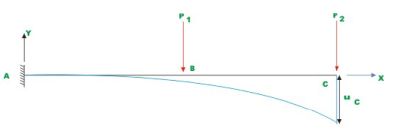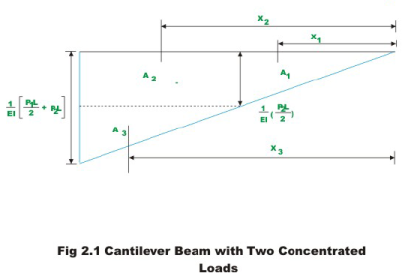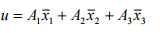(2.1)

where u is the tangential deviation of point C with respect to a tangent at A . Since, in this case the tangent at A is horizontal, the tangential deviation of point C is nothing but the vertical deflection at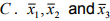are the distances from point C to the centroids of respective areas respectively.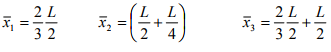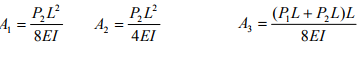Hence,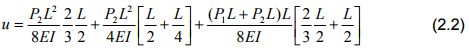After simplification one can write,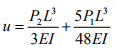(2.3)

Now consider the forces being applied separately and evaluate deflection at C in each of the case.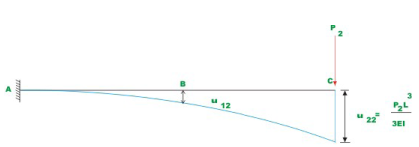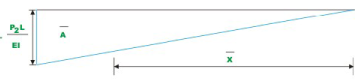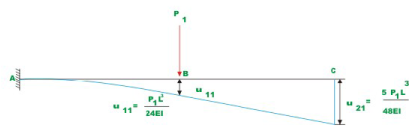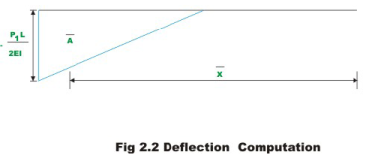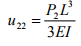(2.4)

where u22 is deflection at C (2) when load P1 is applied at C(2) itself. And,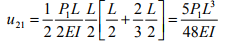(2.5)

where u21 is the deflection at C(2) when load is applied at B(1) . Now the total deflection at C when both the loads are applied simultaneously is obtained by adding u22 and u21.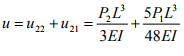(2.6)

Hence it is seen from equations (2.3) and (2.6) that when the structure behaves linearly, the total deflection caused by forces P1, P2,......Pn at any point in the structure is the sum of deflection caused by forces P1, P2,......Pn acting independently on the structure at the same point. This is known as the Principle of Superposition. The method of superposition is not valid when the material stress-strain relationship is non-linear. Also, it is not valid in cases where the geometry of structure changes on application of load. For example, consider a hinged-hinged beam-column subjected to only compressive force as shown in Fig. 2.3(a). Let the compressive force P be less than the Euler’s buckling load of the structure. Then deflection at an arbitrary point C (say)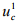is zero. Next, the same beamcolumn be subjected to lateral load Q with no axial load as shown in Fig. 2.3(b). Let the deflection of the beam-column at C be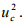Now consider the case when the same beam-column is subjected to both axial load P and lateral load Q. As per the principle of superposition, the deflection at the centre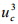must be the sum of deflections caused by P and Q when applied individually. However this is not so in the present case. Because of lateral deflection caused by Q, there will be additional bending moment due to P at C. Hence, the net deflectionwill be more than the sum of deflectionsand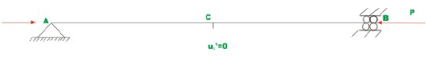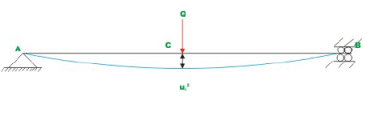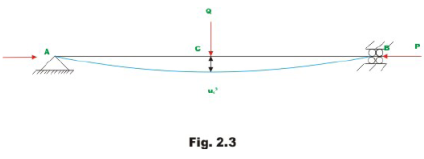Strain Energy

Consider an elastic spring as shown in the Fig.2.4. When the spring is slowly pulled, it deflects by a small amount u1. When the load is removed from the spring, it goes back to the original position. When the spring is pulled by a force, it does some work and this can be calculated once the load-displacement relationship is known. It may be noted that, the spring is a mathematical idealization of the rod being pulled by a force  P axially. It is assumed here that the force is applied gradually so that it slowly increases from zero to a maximum value P. Such a load is called static loading, as there are no inertial effects due to motion. Let the load-displacement relationship be as shown in Fig. 2.5. Now, work done by the external force may be calculated as,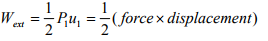(2.7)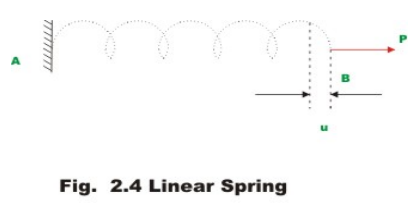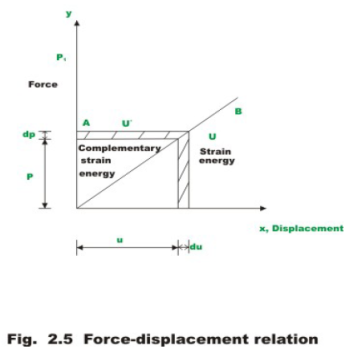The area enclosed by force-displacement curve gives the total work done by the externally applied load. Here it is assumed that the energy is conserved i.e. the work done by gradually applied loads is equal to energy stored in the structure. This internal energy is known as strain energy. Now strain energy stored in a spring is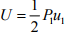(2.8)

Work and energy are expressed in the same units. In SI system, the unit of work and energy is the joule (J), which is equal to one Newton metre (N.m). The strain energy may also be defined as the internal work done by the stress resultants in moving through the corresponding deformations. Consider an infinitesimal element within a three dimensional homogeneous and isotropic material. In the most general case, the state of stress acting on such an element may be as shown in Fig. 2.6. There are normal stresses (σx, σy and σz) and shear stresses (τxy, τyz, and τzx) acting on the element. Corresponding to normal and shear stresses we have normal and shear strains. Now strain energy may be written as,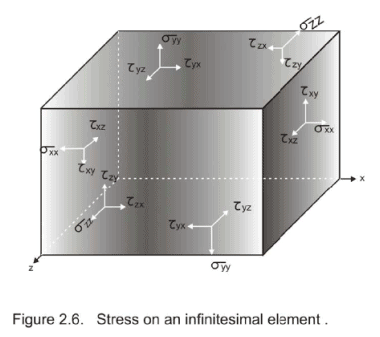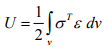(2.9)

in which στ  is the transpose of the stress column vector i.e.,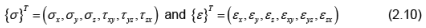The strain energy may be further classified as elastic strain energy and inelastic strain energy as shown in Fig. 2.7. If the force P is removed then the spring shortens. When the elastic limit of the spring is not exceeded, then on removal of load, the spring regains its original shape. If the elastic limit of the material is exceeded, a permanent set will remain on removal of load. In the present case, load the spring beyond its elastic limit. Then we obtain the load-displacement curve OABCDO as shown in Fig. 2.7. Now if at B, the load is removed, the spring gradually shortens. However, a permanent set of OD is till retained. The shaded area BCD is known as the elastic strain energy. This can be recovered upon removing the load. The area OABDO represents the inelastic portion of strain energy.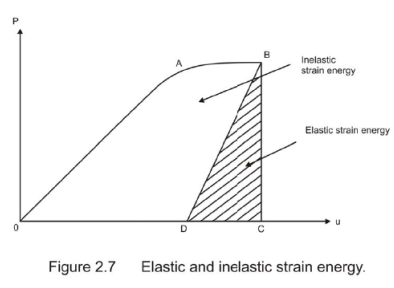The area OABCDO corresponds to strain energy stored in the structure. The area OABEO is defined as the complementary strain energy. For the linearly elastic structure it may be seen that

Area OBC = Area OBE

i.e. Strain energy = Complementary strain energy

This is not the case always as observed from Fig. 2.7. The complementary energy has no physical meaning. The definition is being used for its convenience in structural analysis as will be clear from the subsequent chapters.

Usually structural member is subjected to any one or the combination of bending moment; shear force, axial force and twisting moment. The member resists these external actions by internal stresses. In this section, the internal stresses induced in the structure due to external forces and the associated displacements are calculated for different actions. Knowing internal stresses due to individual forces, one could calculate the resulting stress distribution due to combination of external forces by the method of superposition. After knowing internal stresses and deformations, one could easily evaluate strain energy stored in a simple beam due to axial, bending, shear and torsional deformations.

Offer running on EduRev: Apply code STAYHOME200 to get INR 200 off on our premium plan EduRev Infinity!

## Structural Analysis

30 videos|122 docs|28 tests

,

,

,

,

,

,

,

,

,

,

,

,

,

,

,

,

,

,

,

,

,

,

,

,

;